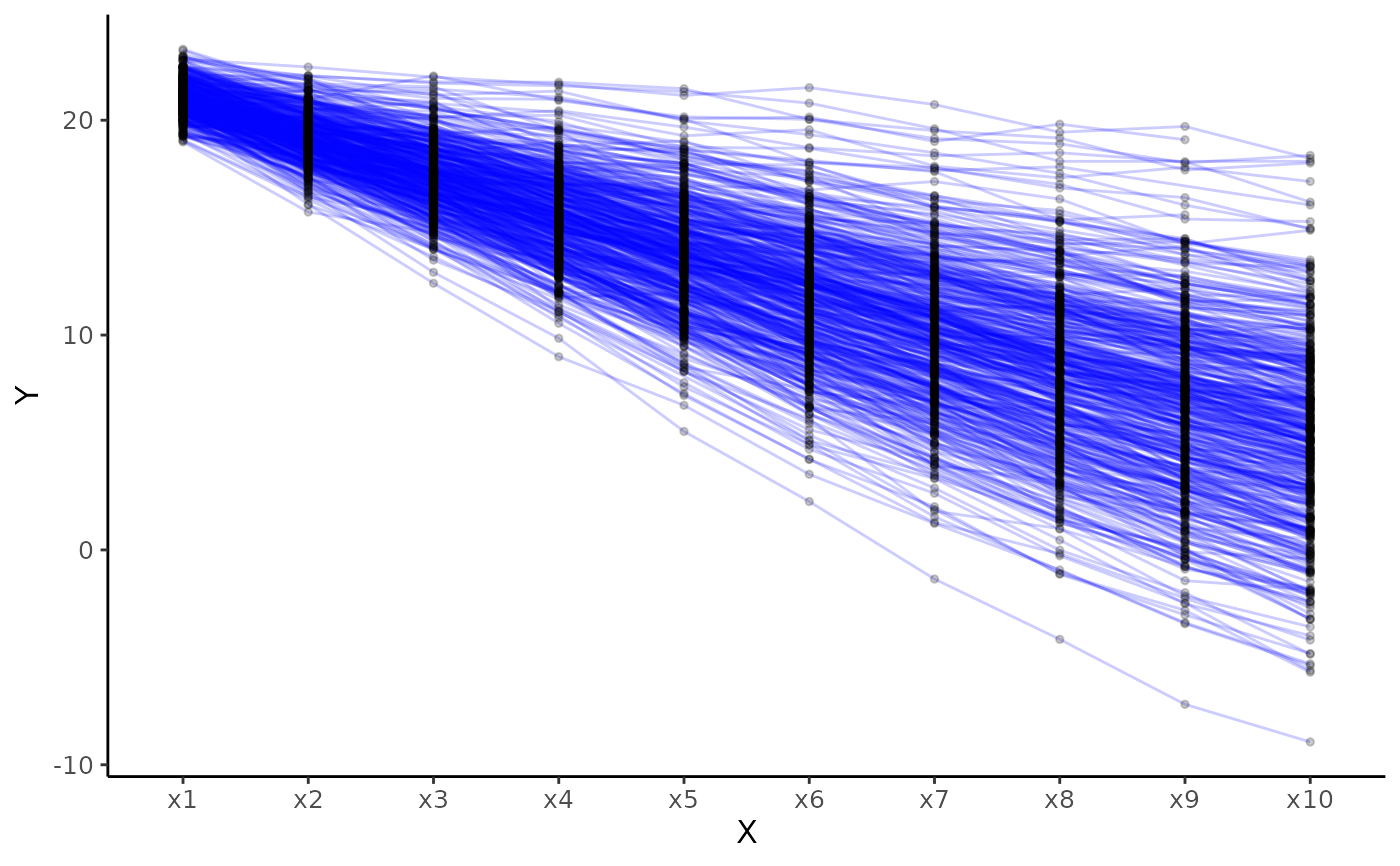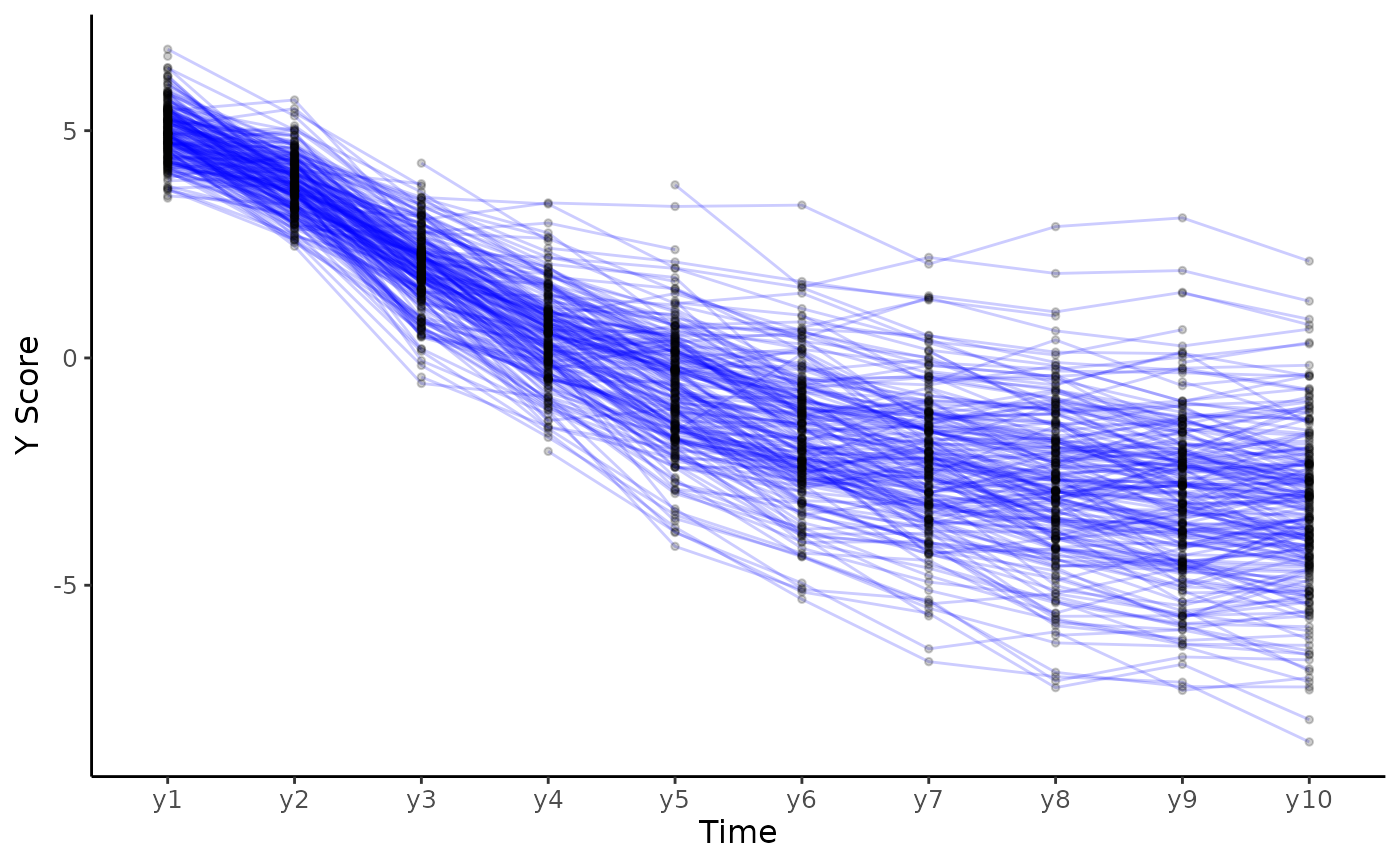Plot individual trajectories

plot_trajectories(
data,
id_var,
var_list,
line_colour = "blue",
group_var = NULL,
point_colour = "black",
line_alpha = 0.2,
point_alpha = 0.2,
point_size = 1,
smooth = FALSE,
smooth_method = "loess",
smooth_se = FALSE,
xlab = "X",
ylab = "Y",
scale_x_num = FALSE,
scale_x_num_start = 1,
random_sample_frac = 1,
seed = 1234,
title_n = FALSE,
connect_missing = TRUE
)

## Arguments

data Dataset in wide format. String, specifying id variable. Vector, specifying variable names to be plotted in sequential order. String, specifying colour of lines. String, specifying variable name of group, each group will get individual colour lines. This overwrites the line_colour argument. Also consider other options to look at trajectories like facet_wrap which may be more appropriate. String, specifying, colour of points. Numeric, specifying alpha of lines. Numeric, specifying alpha of points. Numeric, size of point Logical, add smoothed conditional means using geom_smooth. String, specifying method to be used for calculating average line, see geom_smooth. Logical, specifying whether to add standard error of average line or not. String for x axis label. String for y axis label. Logical, if TRUE print sequential numbers starting from 1 as x axis labels, if FALSE use variable names. Numeric, if scale_x_num = TRUE this is the starting value of the x axis. The fraction of rows to select (from wide dataset), default is set to 1 (100 percent) of the sample. Set seed for random sample if random_sample_frac argument is used. Logical, specifying whether to print title with number and percentage of cases used for the plot. Logical, specifying whether to connect points by id_var across missing values.

ggplot2 object

## Examples

# Create plot for construct x
plot_trajectories(data = data_bi_lcsm,
id_var = "id",
var_list = c("x1", "x2", "x3", "x4", "x5",
"x6", "x7", "x8", "x9", "x10"))
#> Warning: Removed 260 rows containing missing values (geom_point).# Create plot for construct y specifying some ather arguments
plot_trajectories(data = data_bi_lcsm,
id_var = "id",
var_list = c("y1", "y2", "y3", "y4", "y5",
"y6", "y7", "y8", "y9", "y10"),
xlab = "Time", ylab = "Y Score",
connect_missing = FALSE, random_sample_frac = 0.5)
#> Warning: Removed 58 row(s) containing missing values (geom_path).#> Warning: Removed 270 rows containing missing values (geom_point).